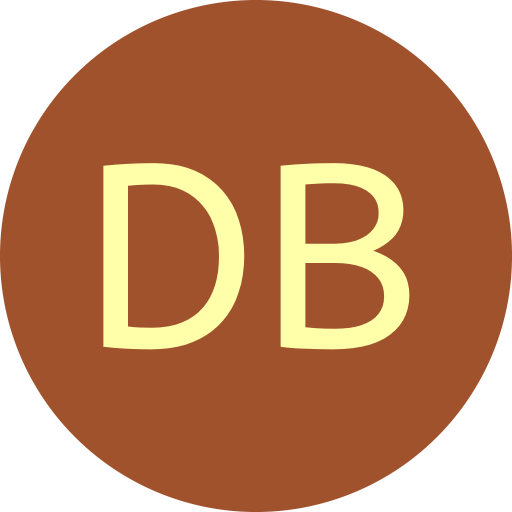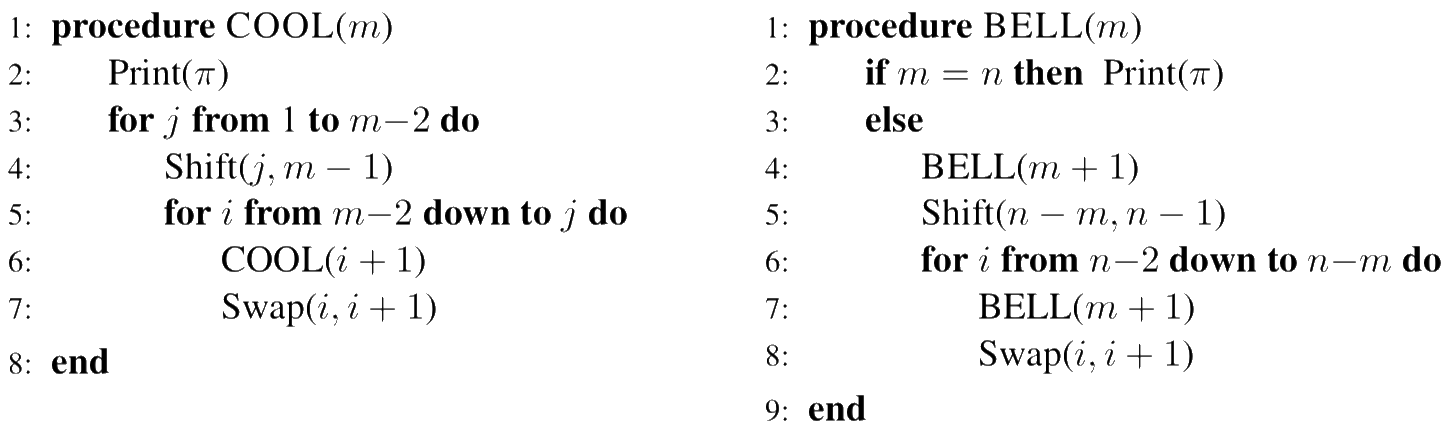#De Bruijn Sequence and Universal Cycle Constructions

Concatenation Method Output
 Algorithm Cool-lex Bell-ringer Order $n$
Constructing universal cycles for shorthand permutations using concatenation approaches

Given a permutation listing $\pi_1, \pi_2, \pi_3, \ldots , \pi_{(n-1)!}$ of permutations of order $n{-}1$, consider the concatenation of these permutations with $n$ prepended to the start of each permutation: $$\color{red}{n}\pi_1 \ \cdot \ \color{red}{n}\pi_2 \ \cdot \ \color{red}{n}\pi_3 \ \cdots \ \color{red}{n}\pi_{(n-1)!}$$ Amazingly, when the permutations are listed in either cool-lex or bell-ringer order, the above string is a universal cycle for shorthand permutations. Exact conditions for when such a permutation listing yields a universal cycle are known [HRW12]. By applying the permutation generation algorithms given below, the corresponding universal cycles can be generated in $O(1)$-time per symbol.

Examples of cool-lex order and bell-ringer order

Cool-lex order for $n=4$: $$4321, 3214, 2134, 1234, 2314, 3124, 1324, 3241, 2341, 3421, 4213, 2143,$$ $$1243, 2413, 4123, 1423, 4231, 2431, 4312, 3142, 1342, 3412, 4132, 1432.$$

Bell-ringer order for $n=4$: $$4321, 3214, 3241, 3421, 4213, 2134, 2143, 2413, 4231, 2314, 2341, 2431,$$ $$4312, 3124, 3142, 3412, 4123, 1234, 1243, 1423, 4132, 1324, 1342, 1432.$$

The corresponding universal cycles for shorthand permutations of order $n=5$ are respectively: $$\color{red}{5}4321\color{red}{5}3214\color{red}{5}2134\color{red}{5}1234\color{red}{5}2314\color{red}{5}3124\color{red}{5}1324\color{red}{5}3241\color{red}{5}2341\color{red}{5}3421\color{red}{5}4213\color{red}{5}2143$$ $$\color{red}{5}1243\color{red}{5}2413\color{red}{5}4123\color{red}{5}1423\color{red}{5}4231\color{red}{5}2431\color{red}{5}4312\color{red}{5}3142\color{red}{5}1342\color{red}{5}3412\color{red}{5}4132\color{red}{5}1432$$ and $$\color{red}{5}4321\color{red}{5}3214\color{red}{5}3241\color{red}{5}3421\color{red}{5}4213\color{red}{5}2134\color{red}{5}2143\color{red}{5}2413\color{red}{5}4231\color{red}{5}2314\color{red}{5}2341\color{red}{5}2431$$ $$\color{red}{5}4312\color{red}{5}3124\color{red}{5}3142\color{red}{5}3412\color{red}{5}4123\color{red}{5}1234\color{red}{5}1243\color{red}{5}1423\color{red}{5}4132\color{red}{5}1324\color{red}{5}1342\color{red}{5}1432.$$

An interesting question is whether there exists other simple concatenation constructions.

#### Algorithms to list permutations in cool-lex and bell-ringer order

The following simple recursive algorithms [HRW12] list permutations of order $n{-}1$ in cool-lex or bell-ringer order, where:

• the current permutation $\pi = p_1p_2\cdots p_{n-1}$ is initialized to $(n{-}1)\cdots 321$,
• the function Swap($i,j$) transposes the elements $p_i$ and $p_j$,
• the function Shift($i,j$) replaces $p_i\cdots p_j$ with $p_{i+1}\cdots p_jp_i$.
The initial calls to generate the listings are COOL($n$) and BELL(2).Note: the above algorithm COOL fixes a typo in the original paper [HRW12] replacing COOL($i$) with COOL($i{+}1$).